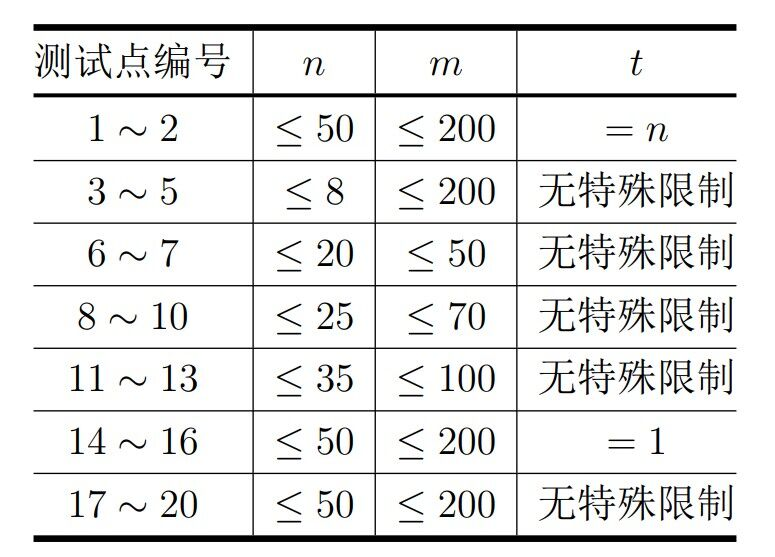# #804. 【统一省选2023】染色数组

1. 每个数 $A_{i}$ 要么被染成红色，要么被染成绿色。
2. 红色的数从左到右依次严格递增，绿色的数从左到右依次严格递减。

1. 如果 $A_{j}$ 染成红色，且 $A_{j} < A_{i}$，则该元素对得 $m-A_{j}+1$ 分;
2. 如果 $A_{j}$ 染成绿色，且 $A_{j} > A_{i}$，则该元素对得 $A_{j}$ 分;
3. 不满足 1 或 2 ，则该元素对得 $0$ 分。

• 第一问：有多少种确定 $A$ 中后 $n-t$ 个数的方案使得 $A$ 是一个完美数组？
• 第二问：所有可能的完美数组的得分和是多少？

### 输出格式

• 第一个整数表示优秀数组的数量模 $998244353$ 的值；

• 第二个整数表示优秀数组的得分之和模 $998244353$ 的值。

### 样例一

#### input

5
6 10 6
1 9 3 4 7 6
5 8 4
1 7 2 6
9 10 2
3 6
6 11 6
1 7 5 8 3 9
9 10 5
5 10 6 4 7

#### output

1 63
8 245
29378 1267731
1 17
78 1820

### 数据范围